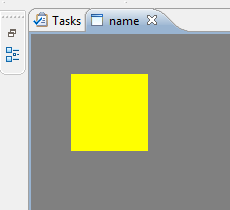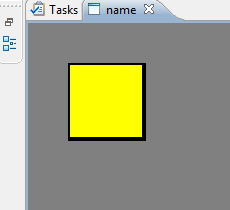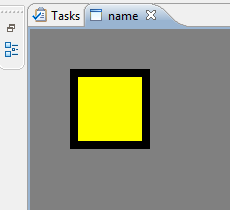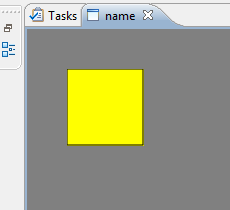# Eclipse Draw2D Border Magic

While using Eclipse Draw2D, I stumbled over some quite interesting behavior on win32 using rectangles and scaling.

We have a scalable layer and added a rectangle. When the layer was actually scaling (so not 1.0) strange artifacts appeared on win32 platforms. Finally it turned out that it was a negative line size with the outline flag set to true. Which renders on Linux as if outline would be set to false. Yet interesting was that setting the lineSize to 0.0 (zero) while keeping the outline flag true causes a hairline to be drawn. So a line which is 1 pixel, independent of the scale factor.

SettingsScreenshot
``````figure.setOutline ( false );
````````````figure.setOutline ( true );
``````

Which is the same as:

``````figure.setOutline ( true );
figure.setLineWidthFloat ( 1.0f );
````````````figure.setOutline ( true );
figure.setLineWidthFloat ( 0.0f );
``````

This is the actual hairline variant.``````figure.setOutline ( true );
figure.setLineWidthFloat ( 2.0f );
````````````figure.setOutline ( true );
figure.setLineWidthFloat ( -1.0f );
``````

Note the small artefacts at on the sides. These are invisible if run under Linux.The full code to create the canvas was:

``````ScalableLayeredPane pane = new ScalableLayeredPane();

Layer layer = new Layer();
layer.setLayoutManager(new StackLayout());

Figure figure = new Figure ();
figure.setLayoutManager(new XYLayout());
figure.setBackgroundColor(Display.getDefault().getSystemColor(SWT.COLOR_DARK_GRAY));
figure.setOpaque(true);

RectangleFigure r = new RectangleFigure();
r.setBackgroundColor(Display.getDefault().getSystemColor(SWT.COLOR_YELLOW));